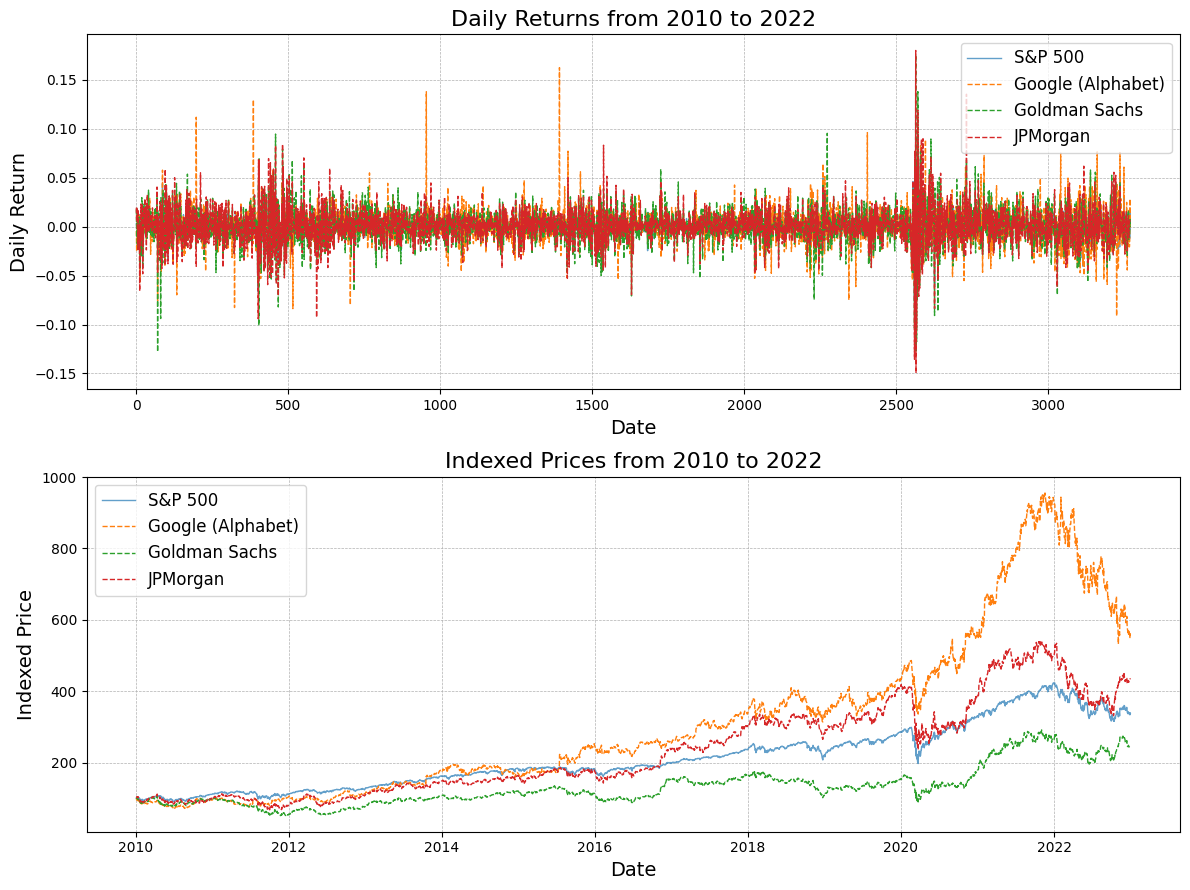# SRISK#

## Introduction#

A conditional capital shortfall measure of systemic risk by Brownlees and Engle (2017).

### Capital Shortfall#

Capital shortfall is a firm’s required capital reserve minus the firm’s equity. Specifically, capital shortfall of a firm $$i$$ on day $$t$$ is

(1)#$CS_{it} = kA_{it} - W_{it} = k(D_{it}+W_{it}) - W_{it}$

where,

• $$W_{it}$$ is the market value of equity

• $$D_{it}$$ is the book value of debt

• $$A_{it} = W_{it} + D_{it}$$ is the value of quasi assets

• $$k$$ is the prudential capital fraction, set to 8%

A positive capital shortfall $$CS$$ means the firm is in distress, i.e., the capital reserve required is larger than the firm’s equity value.

### Systemic Event and SRISK#

A systemic event is a market decline below a threshold $$C$$ over a time horizon $$h$$.

If the multiperiod arithmetic market return between $$t+1$$ to $$t+h$$ is $$R_{mt+1:t+h}$$, then the systemic event is $$\{R_{mt+1:t+h}<C\}$$.

Note

$$h=1$$ month and $$C=-10\%$$ are chosen in Brownlees and Engle (2017).

SRISK of a firm $$i$$ is its expected capital shortfall conditional on a systemic event.

(2)#$SRISK_{it} = E_t(CS_{it+h} | R_{mt+1:t+h} < C)$

The total amount of systemic risk in the financial system is measured as the sum of all firm-level SRISK of the $$N$$ institutions in the system with positive SRISK measures.

(3)#$SRISK_{t} = \sum_{i=1}^{N} SRISK_{it}$

Note

Institutions with negative SRISK are ignored. In a crisis it is unlikely that surplus capital will be easily mobilized through mergers or loans to support failing firms.

### Computation of SRISK#

First, we expand $$CS_{it+h}$$,

(4)#\begin{split}\begin{align*} SRISK_{it} &= E_t(CS_{it+h} | R_{mt+1:t+h} < C) \\\\ &= k E_t(D_{it+h} | R_{mt+1:t+h} < C) + (1-k) E_t(W_{it+h} | R_{mt+1:t+h} < C) \end{align*}\end{split}

If debt cannot be renegotiated in case of systemic event,

(5)#$E_t(D_{it+h} | R_{mt+1:t+h} < C)=D_{it}$

So we have,

(6)#\begin{split}\begin{align*} SRISK_{it} &= k D_{it} + (1-k) W_{it} (1 - LRMES_{it}) \\\\ &= W_{it} [k LVG_{it} + (1-k) LRMES_{it} - 1] \end{align*}\end{split}

where,

Important

The key step in computing SRISK is estimating the Long-Run Marginal Expected Shortfall (LRMES).

$$LRMES_{it}$$ for firm $$i$$ at time $$t$$ is then defined as

$LRMES_{it} = -E_i[R_{it+1:t+h} | R_{mt+1:t+h} < C]$

Refer to Long-Run Marginal Expected Shortfall (LRMES) for the steps of estimating LRMES using GJR-GARCH(1,1) - DCC.

## API#

class frds.measures.SRISK(firm_returns: ndarray, market_returns: ndarray, W: float | ndarray, D: float | ndarray)[source]#

SRISK of firm(s) or market at a given time

__init__(firm_returns: ndarray, market_returns: ndarray, W: float | ndarray, D: float | ndarray) None[source]#
Parameters:
• firm_returns (np.ndarray) – (n_days,) array of firm log returns. Can also be (n_day,n_firms) array of multiple firms’ log returns.

• market_returns (np.ndarray) – (n_days,) array of market log returns

• W (float | np.ndarray) – market value of equity. It can be either a single float value for a firm or a (n_firms,) array for multiple firms.

• D (float | np.ndarray) – book value of debt. It can be either a single float value for a firm or a (n_firms,) array for multiple firms.

Note

If firm_returns is a (n_day,n_firms), then W and D must be of shape (n_firms,).

estimate(k=0.08, lrmes_h=22, lrmes_S=10000, lrmes_C=-0.1, lrmes_random_seed=42, aggregate_srisk=False) ndarray | float[source]#
Parameters:
• k (float, optional) – prudential capital factor. Defaults to 8%.

• lrmes_h (int, optional) – parameter used to estimate lrmes(). Prediction horizon. Defaults to 22.

• lrmes_S (int, optional) – parameter used to estimate LRMES. The number of simulations. Defaults to 10_000.

• lrmes_C (float, optional) – parameter used to estimate LRMES. The markdown decline that defines a systemic event. Defaults to -0.1.

• lrmes_random_seed (int, optional) – random seed in estimating LRMES. Defaults to 42.

• aggregate_srisk (bool, optional) – whether to compute the aggregate SRISK. Defaults to False.

Returns:

If aggregate_srisk=False, (n_firms,) array of firm-level SRISK measures. Otherwise, a single float value for aggregate SRISK.

Return type:

np.ndarray | float

## Examples#

>>> from frds.datasets import StockReturns
>>> returns = StockReturns.stocks_us


frds.datasets.StockReturns.stocks_us provides daily stock returns of a few U.S. stocks, including Google, Goldman Sachs, JPMorgan, and the S&P500 index, from 2010 to 2022.

>>> returns.head()
GOOGL        GS       JPM     ^GSPC
Date
2010-01-05 -0.004404  0.017680  0.019370  0.003116
2010-01-06 -0.025209 -0.010673  0.005494  0.000546
2010-01-07 -0.023280  0.019569  0.019809  0.004001
2010-01-08  0.013331 -0.018912 -0.002456  0.002882
2010-01-11 -0.001512 -0.015776 -0.003357  0.001747
>>> len(returns)
3271


Below is a visualization of the returns and indexed prices.Let’s estimate some SRISKs. I’ll use the last 600 days as the training sample.

>>> gs = returns["GS"].to_numpy()[-600:]
>>> jpm = returns["JPM"].to_numpy()[-600:]
>>> sp500 = returns["^GSPC"].to_numpy()[-600:]


We can estimate the SRISK for Goldman Sachs, assuming it has a market value equity of 100 and debt value of 900.

>>> from frds.measures import SRISK
>>> srisk = SRISK(gs, sp500, W=100.0, D=900.0)
>>> srisk.estimate()
-11.032087743990681


Negative SRISK! So Goldman Sachs with the assumed equity/debt is safe. What if we define a “systemic event” to be a market decline of 5% instead, and assume a even higher leverage?

>>> srisk = SRISK(gs, sp500, W=100.0, D=1500.0)
>>> srisk.estimate(lrmes_C=-0.05)
33.462929665773935


Well, in this extreme case where the bank has a equity to debt ratio of 1/15, and a systemic event defined as market decline of 5% over 22 days, the SRISK of the bank is positive suggesting a capital shortfall.